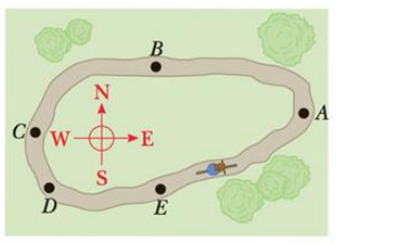Chapter 7, Problem 12CQ

Chapter
Section
Textbook Problem

A child is practicing for a BMX race. His speed remains constant as he goes counterclockwise around a level track with two nearly straight sections and two nearly semicircular sections, as shown in the aerial view of Figure CQ7.12. (a) What are the directions of his velocity at points A, B, and C? For each point, choose one: north, south, east, west, or nonexistent, (b) What are the directions of his acceleration at points A, B, and C?Figure CQ7.12

(a)

To determine

The direction of child’s velocity at A, B and C.

Explanation

Given Info:

The child is moving in anticlockwise direction.

In the point A, the child is moving in the direction from south to north. So, the direction of velocity of the child at the point A is north.

In the point B, the child is moving in the direction from east to west. So, the direction of velocity of the child at the point B is west...

(b)

To determine

The direction of child’s acceleration at A, B and C.

Still sussing out bartleby?

Check out a sample textbook solution.

See a sample solution

The Solution to Your Study Problems

Bartleby provides explanations to thousands of textbook problems written by our experts, many with advanced degrees!

Get Started

Find more solutions based on key concepts